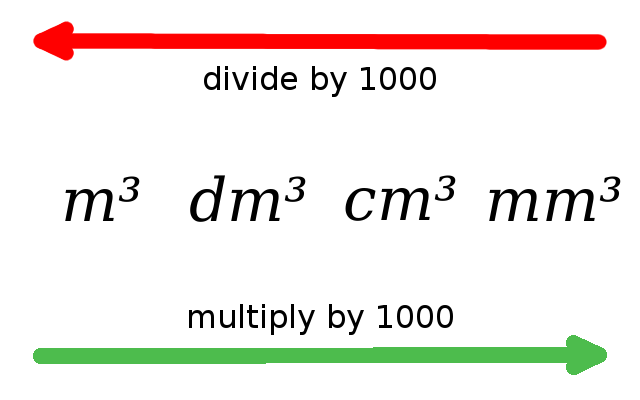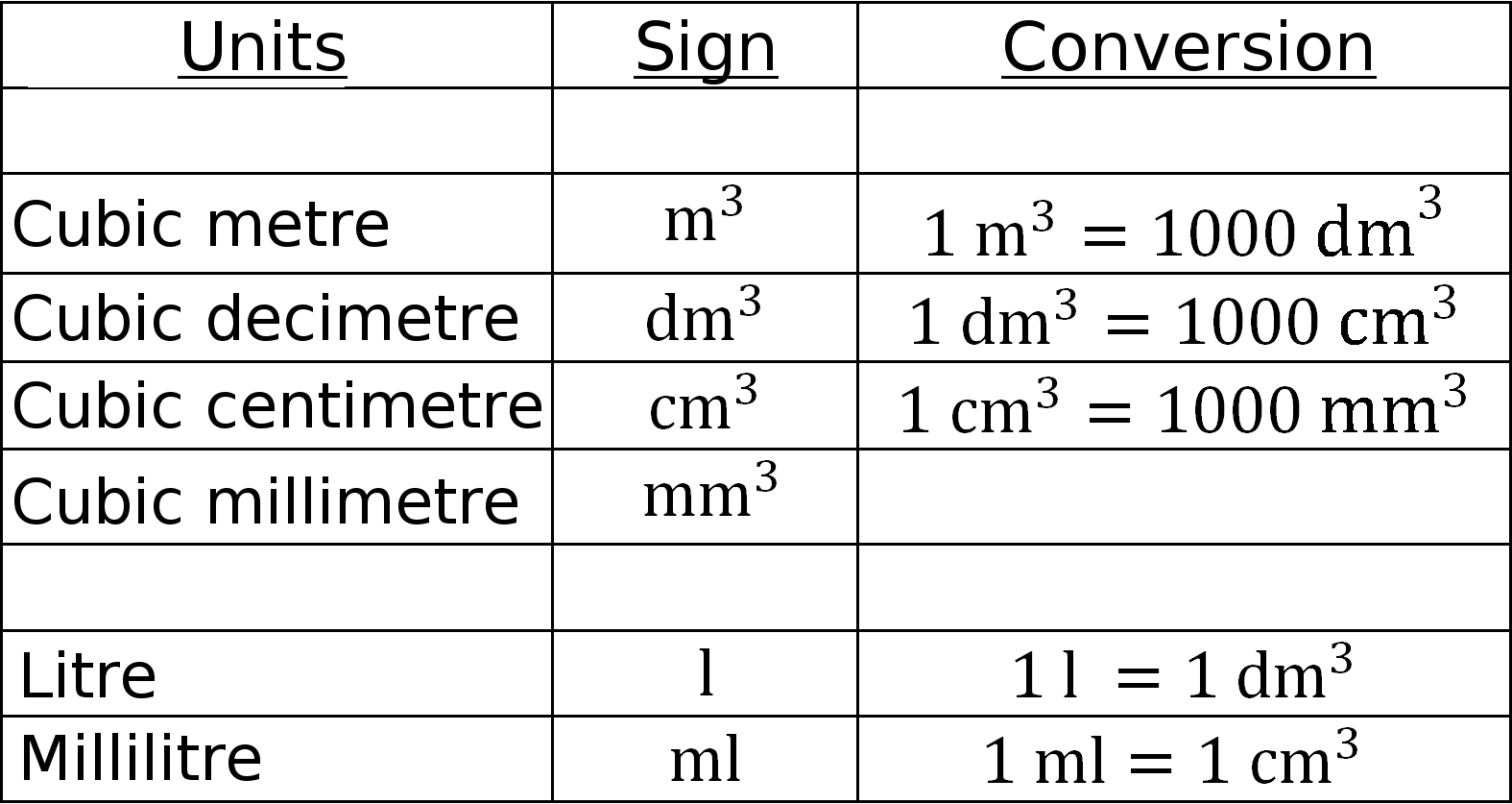Math Geometric formulas Definition volume

# Volume

The volume (formula symbol: $V$) denotes the capacity of a field.
The unit is expressed in cubic (e.g. $m^{ 3 }$).

i

### Hint

Each field has its own formula for calculating the volume. Formulas: see formulas 3D.

### Conversion